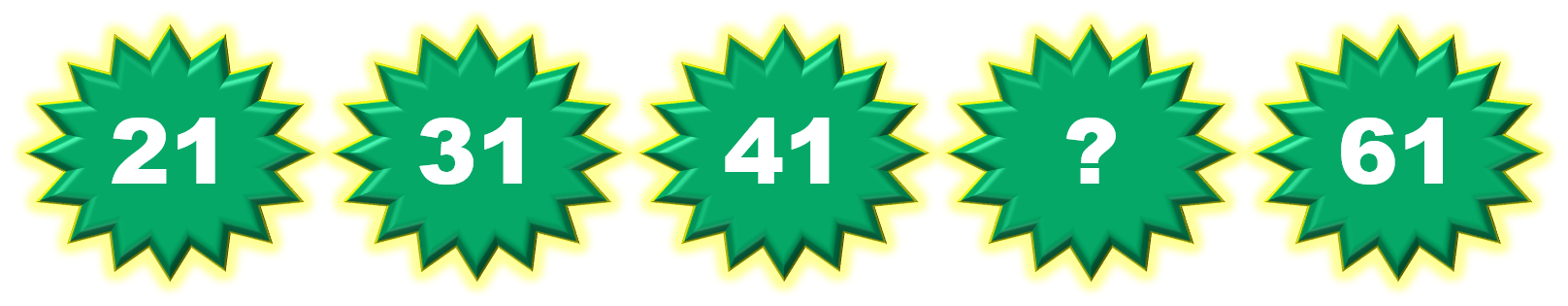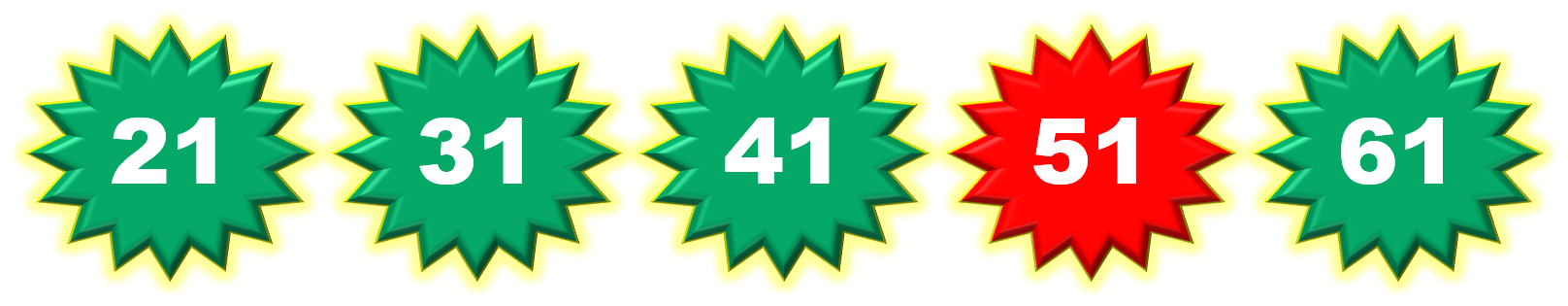1
visibility

Consider the given number pattern and identify the missing number.• A

47

• B

48

• C

50

• D

51

The correct answer is 51.

Solution:

Considering the given number pattern.Here, the numbers are increasing.
So, to find the missing number, we first find the rule.

Note that:
21 + 10 = 31
31 + 10 = 41
41 + 10 = 51
51 + 10 = 61

So, the rule is "add 10".Then, the missing number is 51.